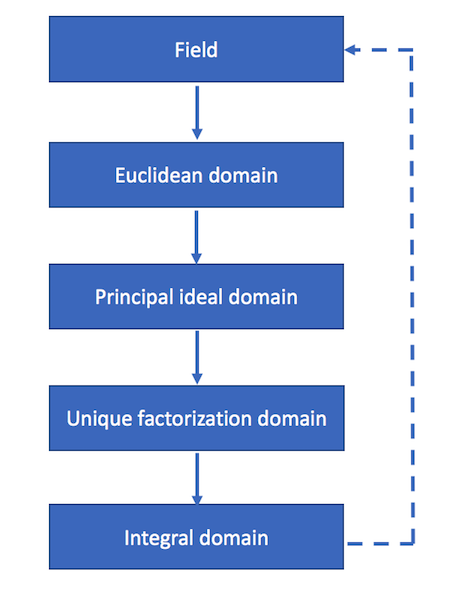# Visualizing kinds of rings

When I first saw ring theory, my impression was that there were dozens of kinds of rings with dozens of special relations between them—more than I could keep up with. In reality, there just a few basic kinds of rings, and the relations between them are simple.

Here’s a diagram that shows the basic kinds of rings and the relations between them. (I’m only looking at commutative rings, and I assume ever ring has a multiplicative identity.)The solid lines are unconditional implications. The dashed line is a conditional implication.

• Every field is a Euclidean domain.
• Every Euclidean domain is a principal ideal domain (PID).
• Every principal ideal domain is a unique factorization domain (UFD).
• Every unique factorization domain is an integral domain.
• A finite integral domain is a field.

Incidentally, the diagram has a sort of embedded pun: the implications form a circle, i.e. a ring.

More mathematical diagrams: